# 【Java学习笔记之九】java二维数组及其多维数组的内存应用拓展延伸

+关注继续查看

int[][] map;
char c[][];

-----------------------------------------------------------------------------------------------------------------------------------------------------------

int[][] m = {{1,2,3},{2,3,4}};

int[][][] b = {{{1,2,3},{1,2,3}},{{3,4,1},{2,3,4}}};

byte[][] b = new byte;
int m[][];
m = new int;

int n[][];
n = new int[]; //只初始化第一维的长度
//分别初始化后续的元素
n = new int;
n = new int;

----------------------------------------------------------------------------------------------------------------------------------------------------------------

---------------------------------------------------------------------------------------------------------------------------------------------------------------

int[][] m = {{1,2,3,1},{1,3},{3,4,2}};
int sum = 0;
for(int i = 0;i < m.length;i++){         //循环第一维下标
sum += m[i].length;                 //第二维的长度相加
}

-----------------------------------------------------------------------------------------------------------------------------------------------------------------

① 需要几维数组
② 每一维的长度是多少
③ 按照怎样的规则存储值
④ 数组值的意义是什么

1 2 3 4
2 3 4 1
3 4 1 2
4 1 2 3

int n = 6;
int[][] arr = new int[n][n];
int data; //数值
//循环赋值
for(int row = 0;row < arr.length;row++){
for(int col = 0;col <arr[row].length;col++){
data = row + col + 1;
if(data <= n){
arr[row][col] = data;
}else{
arr[row][col] = data %n;
}
}
}
//输出数组的值
for(int row = 0;row < arr.length;row++){
for(int col = 0;col <arr[row].length;col++){
System.out.print(arr[row][col]);
System.out.print(' ');
}
System.out.println();
}

-----------------------------------------------------------------------------------------------------------------------------------------------------------------

1
1 1
1 2 1
1 3 3 1
1 4 6 4 1

int[][] arr = new int;
//循环赋值
for(int row = 0;row < arr.length;row++){
for(int col = 0;col <= row;col++){
if(col == 0){ //第一列
arr[row][col] = 1;
}else{
arr[row][col] =arr[row - 1][col] + arr[row - 1][col - 1];
}
}
}
//输出数组的值
for(int row = 0;row < arr.length;row++){
for(int col = 0;col <= row;col++){
System.out.print(arr[row][col]);
System.out.print(' ');
}
System.out.println();
}

-----------------------------------------------------------------------------------------------------------------------------------------------------------------

{
{0,0,0,1,0,0,0},
{0,0,1,0,1,0,0},
{0,1,0,0,0,1,0},
{1,0,0,0,0,0,1},
{0,1,0,0,0,1,0},
{0,0,1,0,1,0,0},
{0,0,0,1,0,0,0}
}

int[][] map = {
{0,0,0,1,0,0,0},
{0,0,1,0,1,0,0},
{0,1,0,0,0,1,0},
{1,0,0,0,0,0,1},
{0,1,0,0,0,1,0},
{0,0,1,0,1,0,0},
{0,0,0,1,0,0,0}
};
//输出数组的值
for(int row = 0;row < map.length;row++){
for(int col = 0;col <map[row].length;col++){
switch(map[row][col]){
case 0:
System.out.print(' ');
break;
case 1:
System.out.print('*');
break;
}
}
System.out.println();
}

------------------------------------------------------------------------------------------------------------------------------------------------------------------

1    2    3    4         1     2     3     4     5
12  13 14   5         14   15  16    17   6
11  16  15  6         13   20  19    18   7
10   9    8   7         12   11  10     9    8
4X4 螺旋数组4X5 螺旋数组对于螺旋数组来说，其中的数值很有规则，就是按照旋转的结构数值每次加1，实现该功能需要对数组和流程控制有角深刻的认识。

int n = 4;
int m = 5;
int[][] data = new int[n][m];
int dire; //当前数字的移动方向
final int UP = 0;             //上
final int DOWN = 1;         //下
final int LEFT = 2;         //左
final int RIGHT = 3;        //右
dire = RIGHT;
int value = 1;             //数组元素的值
int row = 0;                 //第一维下标
int col = 0;                 //第二维下标
data = 1;         //初始化第一个元素
while(value < n * m){
switch(dire){
case UP:
row--; //移动到上一行
if(row < 0){ //超过边界
row++; //后退
dire = RIGHT;
continue; //跳过该次循环
}else if(data[row][col] != 0){//已赋值
row++; //后退
dire = RIGHT;
continue; //跳过该次循环
}
break;
case DOWN:
row++; //移动到下一行
if(row >= n){ //超过边界
row--; //后退
dire = LEFT;
continue; //跳过该次循环
}else if(data[row][col] != 0){//已赋值
row--; //后退
dire = LEFT;
continue; //跳过该次循环
}
break;
case LEFT:
col--; //移动到前一列
if(col < 0){ //超过边界
col++; //后退
dire = UP;
continue; //跳过该次循环
}else if(data[row][col] != 0){//已赋值
col++; //后退
dire = UP;
continue; //跳过该次循环
}
break;
case RIGHT:
col++; //移动到后一行
if(col >= m){ //超过边界
col--; //后退
dire = DOWN;
continue; //跳过该次循环
}else if(data[row][col] != 0){//已赋值
col--; //后退
dire = DOWN;
continue; //跳过该次循环
}
break;
}
value++; //数值增加1
data[row][col] = value;//赋值
}
//输出数组中的元素
for(int i = 0;i < data.length;i++){
for(int j = 0;j < data[i].length;j++){
if(data[i][j] < 10){//右对齐
System.out.print(' ');
}
System.out.print(data[i][j]);
System.out.print(' ');
}
System.out.println();
}

# 二维数组内存结构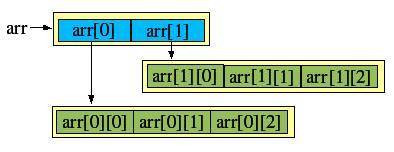# 不规则二维数组

 1 //1.二维数组的定义
2 //2.二维数组的内存空间
3 //3.不规则数组
4 package me.array;
5 public class Array2Demo{
6
7
8     public static void main(String[] args){
9
10         //创建和打印不规则二维数组
11         int arr[ ][ ];
12
13         arr=new int[];//现在说明为不规则数组
14
15         arr=new int;//arr指向另一个一位数组
16         arr=new int;
17         arr=new int;
18
19         //赋值
20         for(int i=0;i<arr.length;i++){
21
22             for(int j=0;j<arr[i].length;j++){
23
24                 arr[i][j]=j;
25             }
26
27         }
28
29         //输出
30         for(int i=0;i<arr.length;i++){
31
32             for(int j=0;j<arr[i].length;j++){
33
34                 System.out.print(arr[i][j]+" ");
35             }
36             System.out.println();
37         }
38
39         /*输出结果：
40         0 1 2 3 4 5 6 7 8 9
41         0 1 2
42         0 1 2 3
43         *///
44
45     }
46
47 }

 1 //3.编写一个方法，返回double型二维数组，数组通过解析字符串参数获得。
2 //如"1,2;3,4,5;6,7,8"
3 //d[0,0]=1.0 d[0,1]=2.0 d[1,0]=3.0 ....
4 package me.parser;
5 public class TestString{
6
7     public static void main(String[] args){
8
9         //1.用字符串分解split(";")成三个字符串数组
10         //2.再分解split(",")
11
12         //声明一个二维数组用来装分解好的字符
13
14
15         String s="1,2;3,4,5;6,7,8";
16         //以split()方法 分解
17         String[] sFirst=s.split(";");
18
19         String[][] word=new String[sFirst.length][];
20
21         int flag=0;
22         for(int i=0;i<sFirst.length;i++){
23
24             //打印出已经分开的
25             //System.out.println(sFirst[i]);
26             /*这条语句输出
27             1,2
28             3,4,5
29             6,7,8
30             *///接下来在按照 ,分开他们放入一个一维数组
31
32             String[] sSecond=sFirst[i].split(",");
33              //~ System.out.println(sSecond.length);
34             //~ /*输出:
35             //~ 2
36             //~ ---------------------------------
37             //~ 3
38             //~ ---------------------------------
39             //~ 3
40             //~ ---------------------------------
41             //~ *///说明每次sSencond这个一维数组的长度不同
42             word[i]=new String[sSecond.length];//这步确定行不规则数组的每行长度
43
44             //为这个数组赋值
45             for(int j=0;j<sSecond.length;j++){
46
47                 word[i][j]=sSecond[j];
48             }
49
50             System.out.println("---------------这是第"+(i+1)+"次循环-------------------");
51         }
52
53         //输出二维数组
54         System.out.println("输出二维数组-------------------");
55         for(int i=0;i<word.length;i++){
56             for(int j=0;j<word[i].length;j++){
57
58                 System.out.print(word[i][j]+" ");
59             }
60             System.out.println();
61         }
62         /*结果：
63         ---------------这是第1次循环-------------------
64         ---------------这是第2次循环-------------------
65         ---------------这是第3次循环-------------------
66         输出二维数组-------------------
67         1 2
68         3 4 5
69         6 7 8
70         输出二维数组-------------------
71         *///
72     }
73 }zookeeper技术分享
5 0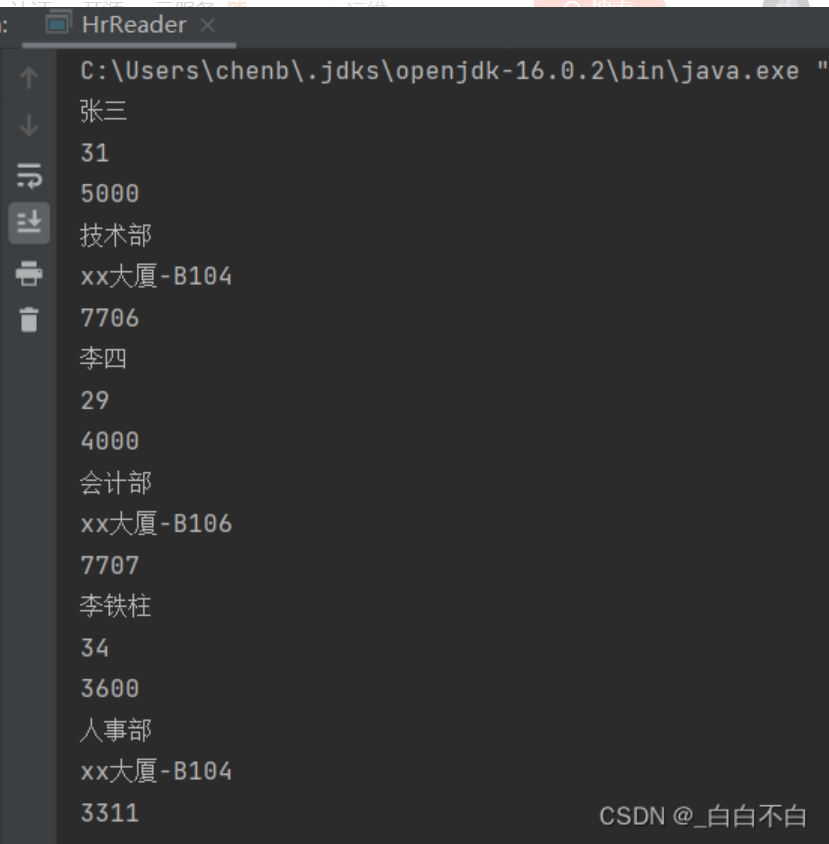【XML入门】一篇文章让你从没听过到熟练运用

6 0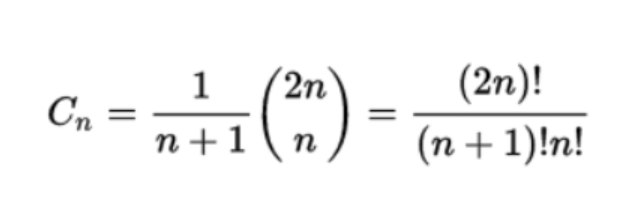【数据结构之栈和队列】熬夜暴肝，有亿点详细

7 06 0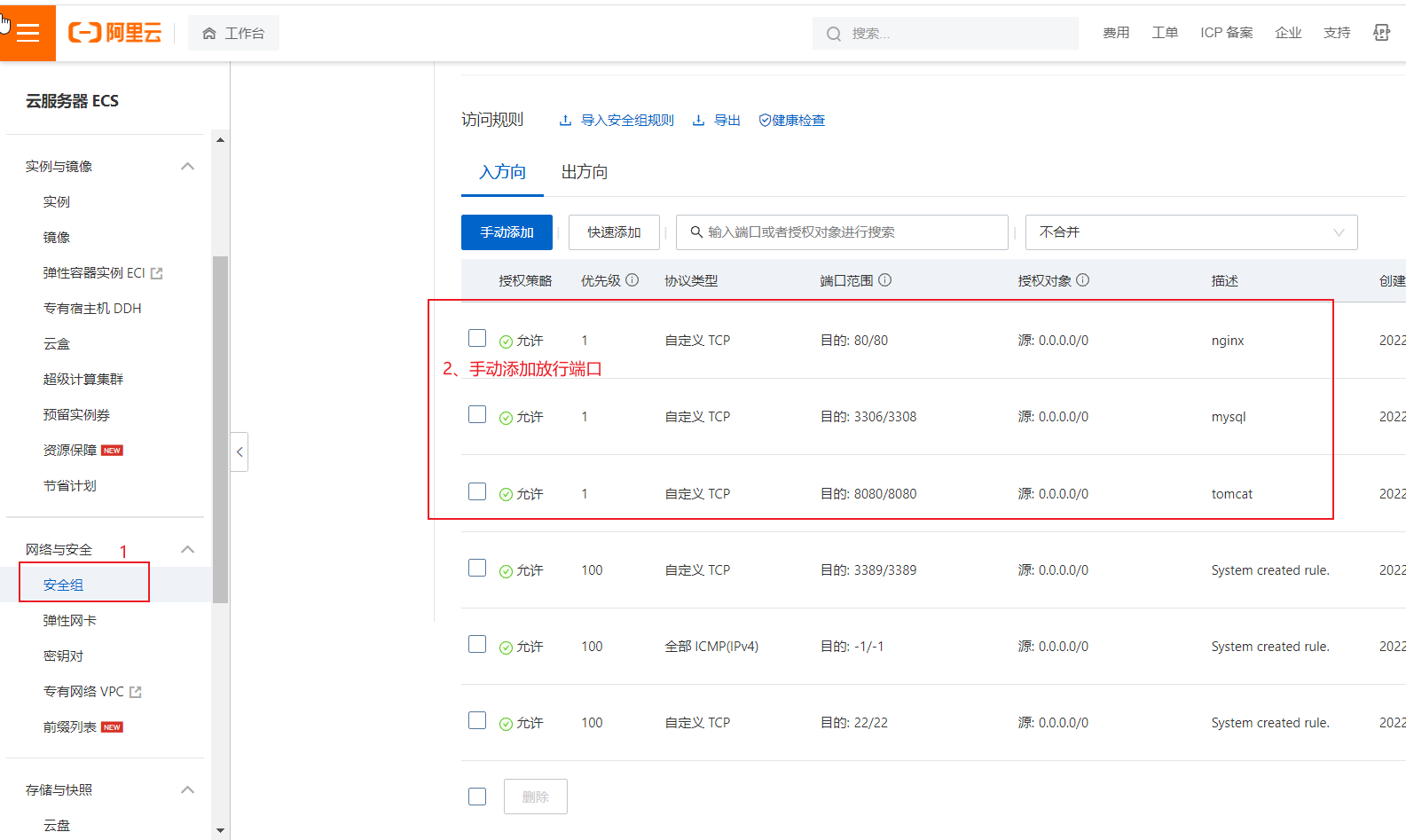4 0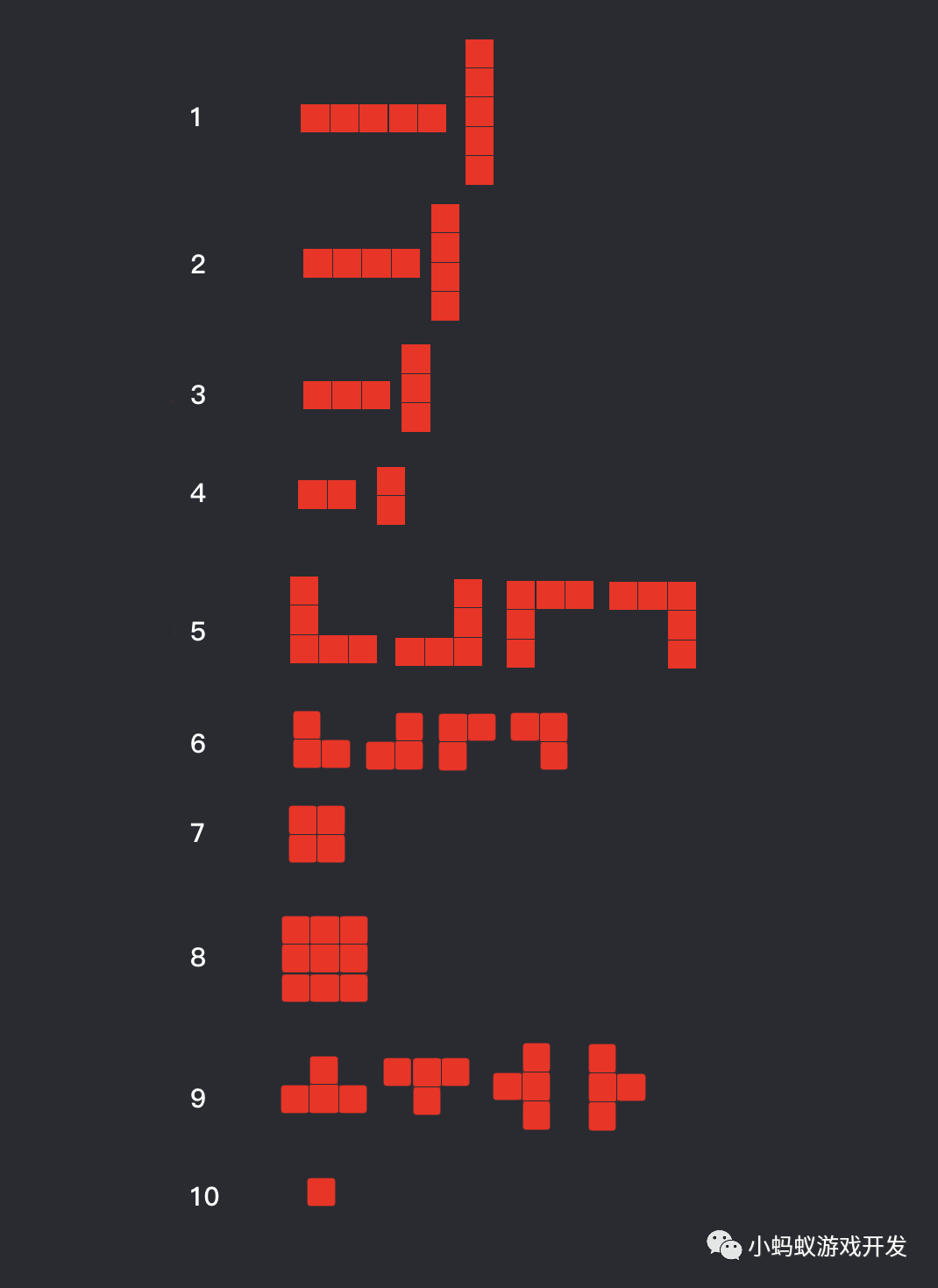**微信小游戏开发实战系列的第13篇，点击上方的#微信小游戏开发实战话题可以查看本系列的所有内容。 本节内容包括：通过使用列表来实现可以设置权重的随机功能，以及游戏中的随机生成形状功能的具体实现。
5 0**这是小蚂蚁游戏开发公众号原创的第71篇。 本文重要内容包含答题类小游戏的制作原理和制作方法，在掌握实现原理和方法后，你也能够根据自己的需要，制作自己的答题类小游戏。
5 0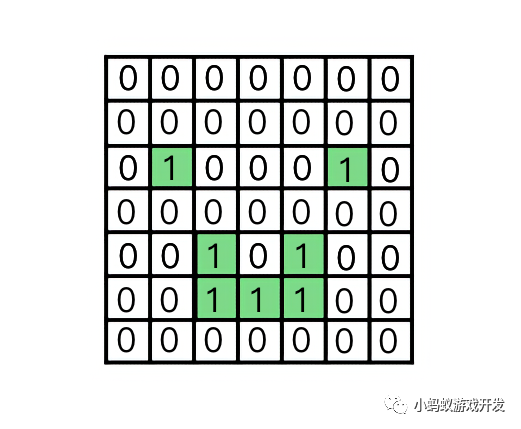**这是微信小游戏开发实战系列的第14篇，点击上方的#微信小游戏开发实战话题可以查看本系列的所有内容。 本文主要内容是介绍精致1010闯关模式的设计和实现思路。
4 0Swift-进阶 13：协议Protocol
Swift-进阶 13：协议Protocol
6 0Swift-进阶 14：泛型
Swift-进阶 14：泛型
6 0
angel_kitty

707

1

OceanBase 入门到实战教程